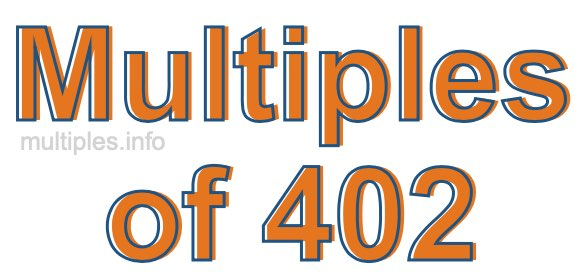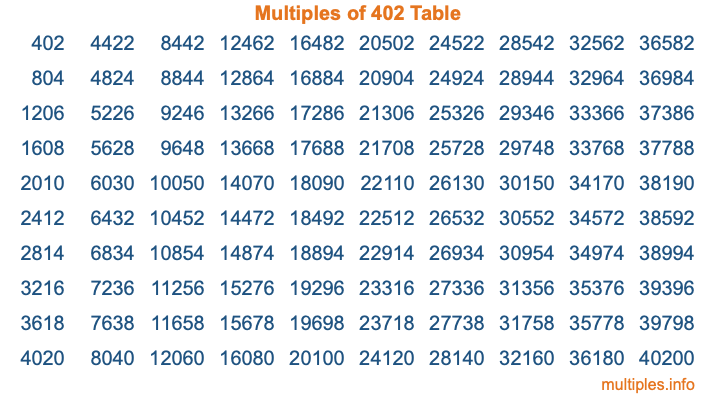Multiples of 402Welcome to the Multiples of 402 page. Here we will first teach you everything you will ever need to know about the multiples of 402, and then give you a study guide summary of everything we taught you to make sure you remember it all. Use this page to look up facts and learn information about the multiples of 402. This page will make you a multiples of four hundred two expert!

Definition of Multiples of 402
Multiples of 402 are all the numbers that when divided by 402 equal an integer. Each of the multiples of 402 are called a multiple. A multiple of 402 is created by multiplying 402 by an integer.

Therefore, to create a list of multiples of 402, you start with 1 multiplied by 402, then 2 multiplied by 402, then 3 multiplied by 402, and so on for as long as you want. Thus, the list of the first five multiples of 402 is 402, 804, 1206, 1608, and 2010. To see a larger list of multiples of 402, see the printable image of Multiples of 402 further down on this page. We also have a category where you can choose any nth multiple of 402.

Multiples of 402 Checker
The Multiples of 402 Checker below checks to see if any number of your choice is a multiple of 402. In other words, it checks to see if there is any number (integer) that when multiplied by 402 will equal your number. To do that, we divide your number by 402. If the the quotient is an integer, then your number is a multiple of 402.

Is  a multiple of 402?

Least Common Multiple of 402 and ...
A Least Common Multiple (LCM) is the lowest multiple that two or more numbers have in common. This is also called the smallest common multiple or lowest common multiple and is useful to know when you are adding our subtracting fractions. Enter one or more numbers below (402 is already entered) to find the LCM.

Check out our LCM Calculator if you need more details about the Least Common Multiple or if you need the LCM for different numbers for adding and subtraction fractions.

nth Multiple of 402
As we stated above, 402 is the first multiple of 402, 804 is the second multiple of 402, 1206 is the third multiple of 402, and so on. Enter a number below to find the nth multiple of 402.

th multiple of 402

Multiples of 402 vs Factors of 402
402 is a multiple of 402 and a factor of 402, but that is where the similarities end. All postive multiples of 402 are 402 or greater than 402. All positive factors of 402 are 402 or less than 402.

Below is the beginning list of multiples of 402 and the factors of 402 so you can compare:

Multiples of 402: 402, 804, 1206, 1608, 2010, etc.

Factors of 402: 1, 2, 3, 6, 67, 134, 201, 402

As you can see, the multiples of 402 are all the numbers that you can divide by 402 to get a whole number. The factors of 402, on the other hand, are all the whole numbers that you can multiply by another whole number to get 402.

It's also interesting to note that if a number (x) is a factor of 402, then 402 will also be a multiple of that number (x).

Multiples of 402 vs Divisors of 402
The divisors of 402 are all the integers that 402 can be divided by evenly. Below is a list of the divisors of 402.

Divisors of 402: 1, 2, 3, 6, 67, 134, 201, 402

The interesting thing to note here is that if you take any multiple of 402 and divide it by a divisor of 402, you will see that the quotient is an integer.

Multiples of 402 Table
Below is an image of the first 100 multiples of 402 in a table. The table is in chronological order, column by column. The first column has the first ten multiples of 402, the second column has the next ten multiples of 402, and so on.The Multiples of 402 Table is also referred to as the 402 Times Table or Times Table of 402. You are welcome to print out our table for your studies.

Negative Multiples of 402
Although not often discussed or needed in math, it is worth mentioning that you can make a list of negative multiples of 402 by multiplying 402 by -1, then by -2, then by -3, and so on, to get the following list of negative multiples of 402:

-402, -804, -1206, -1608, -2010, etc.

Multiples of 402 Summary
Below is a summary of important Multiples of 402 facts that we have discussed on this page. To retain the knowledge on this page, we recommend that you read through the summary and explain to yourself or a study partner why they hold true.

There are an infinite number of multiples of 402.

A multiple of 402 divided by 402 will equal a whole number.

402 divided by a factor of 402 equals a divisor of 402.

The nth multiple of 402 is n times 402.

The largest factor of 402 is equal to the first positive multiple of 402.

402 is a multiple of every factor of 402.

402 is a multiple of 402.

A multiple of 402 divided by a divisor of 402 equals an integer.

402 divided by a divisor of 402 equals a factor of 402.

Any integer times 402 will equal a multiple of 402.

Multiples of a Number
Here you can get the multiples of another number, all with the same attention to detail as we did for multiples of 402 on this page.

Multiples of
Multiples of 403
Did you find our page about multiples of four hundred two educational? Do you want more knowledge? Check out the multiples of the next number on our list!

Copyright  |   Privacy Policy  |   Disclaimer  |   Contact